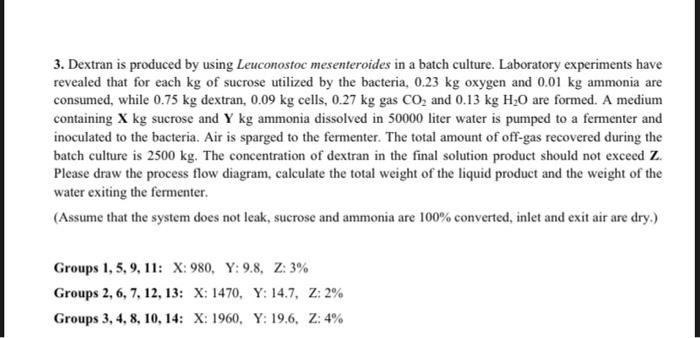Home / Expert Answers / Chemical Engineering / 3-dextran-is-produced-by-using-leuconostoc-mesenteroides-in-a-batch-culture-laboratory-experiment-pa656

# (Solved): 3. Dextran is produced by using Leuconostoc mesenteroides in a batch culture. Laboratory experiment ...3. Dextran is produced by using Leuconostoc mesenteroides in a batch culture. Laboratory experiments have revealed that for each $$\mathrm{kg}$$ of sucrose utilized by the bacteria, $$0.23 \mathrm{~kg}$$ oxygen and $$0.01 \mathrm{~kg}$$ ammonia are consumed, while $$0.75 \mathrm{~kg}$$ dextran, $$0.09 \mathrm{~kg}$$ cells, $$0.27 \mathrm{~kg}$$ gas $$\mathrm{CO}_{2}$$ and $$0.13 \mathrm{~kg} \mathrm{H}_{2} \mathrm{O}$$ are formed. A medium containing $$\mathbf{X} \mathrm{kg}$$ sucrose and $$\mathbf{Y} \mathrm{kg}$$ ammonia dissolved in 50000 liter water is pumped to a fermenter and inoculated to the bacteria. Air is sparged to the fermenter. The total amount of off-gas recovered during the batch culture is $$2500 \mathrm{~kg}$$. The concentration of dextran in the final solution product should not exceed $$\mathbf{Z}$$. Please draw the process flow diagram, calculate the total weight of the liquid product and the weight of the water exiting the fermenter. (Assume that the system does not leak, sucrose and ammonia are 100\% converted, inlet and exit air are dry.) Groups 1, 5, 9, 11: X: 980, Y:9.8, Z: $$3 \%$$ Groups 2,6,7,12, 13: $$\mathrm{X}: 1470, \quad \mathrm{Y}: 14.7, \mathrm{Z}: 2 \%$$ Groups 3,4,8,10,14: X: 1960, Y: 19.6, Z:4\%

We have an Answer from Expert